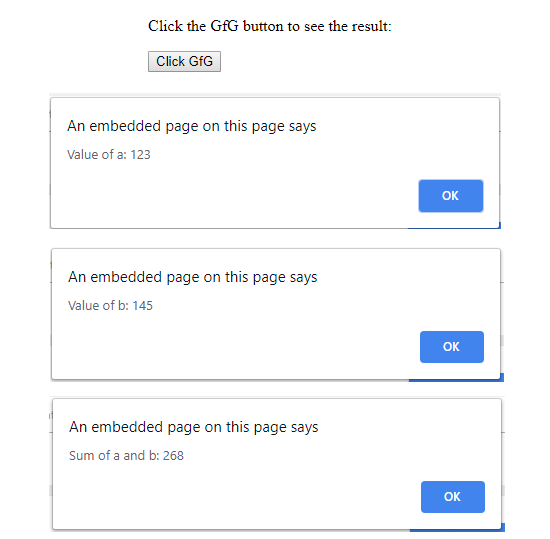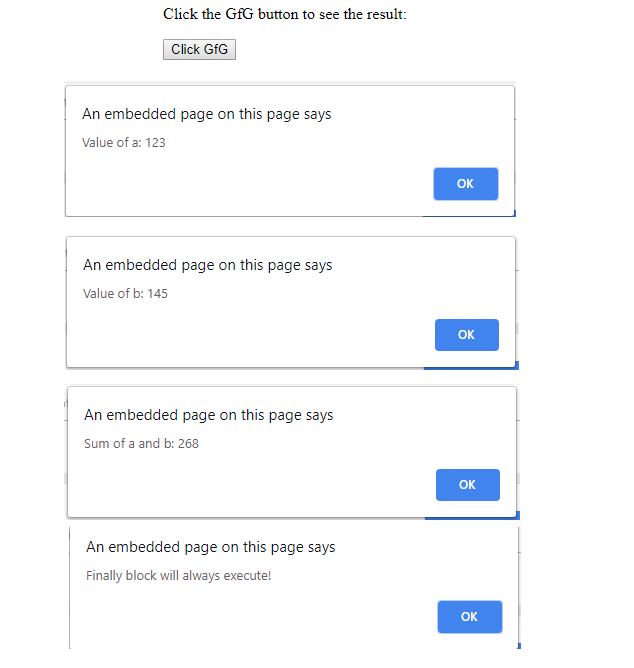# Javascript | Error and Exceptional Handling With Examples

• Difficulty Level : Easy
• Last Updated : 17 Oct, 2018

An error is an action which is inaccurate or incorrect. There are three types of error in programming which are discusses below :

• Syntax error
• Logical error
• Runtime error

Syntax error: According to computer science, a syntax error is an error in the syntax of a sequence of characters or tokens that is intended to be written in a particular programming language or it is also the compile-time error if the syntax is not correct then it will give an error message.

Example:

 ``

As the syntax is not correct of the JavaScript it will affect only the thread that is under this JavaScript and the rest of the code in other threads gets executed as nothing in them depends on the code containing the error.

Logical error: It is the most difficult error to be traced as it is the error on the logical part of the coding or logical error is a bug in a program that causes it to operate incorrectly and terminate abnormally (or crash).

Runtime Error: A runtime error is an error that occurs during the running of the program, also known as the exceptions. In the example that is given below the syntax is correct, but at runtime, it is trying to call a method that does not exist.

Example:

 ``

As in runtime error, there are exceptions and this exception is correct by the help of the try and catch method

try ___ catch method: JavaScript uses the try catch and finally to handle the exception and it also used the throw operator to handle the exception. try have the main code to run and in the catch, give the exception statement all the things that are related to the exception.

Syntax:

 ``

Example 1:

 `<``html``>``    ``<``head``>``        ``<``title``>Error and Exception handling``        ``<``script` `type``=``"text/javascript"``>``            ``function First() {``                ``var a = 123;``                ``var b = 145;``                ``var c = a + b;``                ``alert("Value of a: " + a );``                ``alert("Value of b: " + b );``                ``alert("Sum of a and b: " + c);``            ``}``        ````    ````         ` `    ``<``body``>``        ``<``p``>Click the GfG button to see the result:``         ` `        ``<``form``>``            ``<``input` `type``=``"button"` `value``=``"Click GfG"` `onclick``=``"First();"` `/>``        ````         ` `    ````                    `

Output:In this example, use the finally method which will always execute unconditionally after the try/catch.

Example 2:

 `<``html``>``    ``<``head``>``        ``<``title``>Error and Exception handling``        ``<``script` `type``=``"text/javascript"``>``            ``function First() {``                ``var a = 123;``                ``var b = 145;``                ``var c = a + b;``                ``try {``                    ``alert("Value of a: " + a );``                    ``alert("Value of b: " + b );``                    ``alert("Sum of a and b: " + c);``                ``}``                ``catch ( e ) {``                    ``alert("Error: " + e.description );``                ``}``                ``finally {``                    ``alert("Finally block will always execute!" );``                ``}``            ``}``        ````    ````         ` `    ``<``body``>``        ``<``p``>Click the GfG button to see the result:``         ` `        ``<``form``>``            ``<``input` `type``=``"button"` `value``=``"Click GfG"` `onclick``=``"First();"` `/>``        ````         ` `    ````                    `

Output:My Personal Notes arrow_drop_up